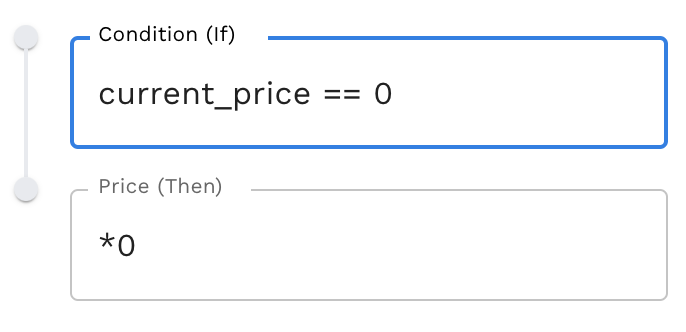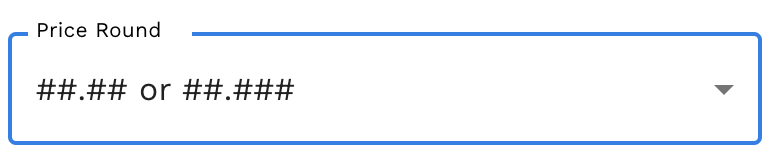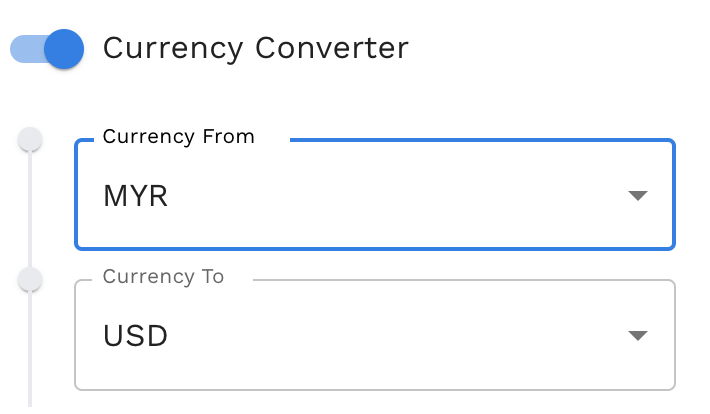# Price Field

Modified on Wed, 06 Dec 2023 at 03:16 PM

```Available on: ✔️ Add ✔️ Update ✔️ Export

If the feed file doesn't have any column as price and you want to apply all the price as 5 for all your products. You can use fixed value to set it. ```There are a few settings for this field which are:

1. Price Condition (Markup)

Price condition is used to add markup or margin to a product's price. The price condition can add a markdown if used for sales.

`Currency Conversion has the first priority compare to the Price Conditions.  `

How to use Price Conditions according to the examples below:Condition (If) Price (Then) Result Showing current_price == 0 *0 If store price = 0Then multiply by 0 (no changes made) any *1.5 All prices will be multiplied by 1.5 or 50% taxable == 1 *1.5 If the taxable is 1, it will be multiplied by 1.5 or 50%(Only for Shopify)1: taxable 0: untaxable any *0 + current_quantity All the prices will be multiplied by 0, and add the current_quantity. The current_quantity value will be taken from the store. col5!="" *0+col5 If the column has any value, then use that column as the price. any *0+(col("price"+80)*1.5 If the stock price wants to add \$80 delivery to it, then add a 50% or 1.5 markup. col_string("Vendor") == "shoe" *1.3 + col("shipping") If the column name vendor has the value shoe, multiply by 1.3 and add the column shipping value to the price.It can be used for any column and is suitable for adding markup based on the conditions. contains("pink", col_string("Description")) *1.1 If the column name Description contains the value of pink from the column, then the price is multiplied by 1.1 or 10%It's used when the value is a string and is not supported by column index. contains("4", col("qty")) *1.5 If the column name qty contains the value of 4 from the column, then the price is multiplied by 1.5 or 50%It's used when the value is numeric and not supported by a column index. price_f > 10 and price_f <= 15 *1.2 If the price is more than 10 and equals or below 15, Then, the price is multiplied by 1.2 or 20%. Use price_f when the comparison values are numeric price_f ==11 *1.5 If the price is 11 Then the price is multiplied by 1.5 or 50% price > col1_f *1 + col1 + col2 If the price is more than column 1, then add column 1 and column 2 to the price. Use col#_f when the comparison values are numeric col5==“shoe” *1.3 If column 5 has the value shoe, then multiply by 1.3It can be used for any column and is suitable for adding markup based on collections price_f < 1 *0 + current_price If the price is less than 1Then it will use the current price in-store (Shopify only).                                           Use price_f when the comparison values are numeric Price ==“high” *1.2 If the price is equal to "high" Then multiply by 1.2                                                                 Use price when comparison values are text any *1.1 All the prices will be multiplied by 1.1 or 10%, which means the price will be discounted by 10% price ==“discount” *1.5 If the price is equal to the "discount"Then it will multiply by 1.5 0r 50%, which markdown the price cost < 40 *1.2 If the cost in the store is less than 40Then multiply the price by 1.2The cost value will be taken from the store. (Shopify only) compare_at_price > 10 *1.5 If compare at a price in the store is more than 10Then multiply the price by 1.5The compare at price value will be taken from the store. (Shopify only) any /0.2 All the prices will be divided by 0.2 col4_date > now *1.2 If the col4 date is more than current_timestampThen multiply by 1.2                                      Use col4_date when the comparison is a date Now refers to current_timestamp col4_date > today *1.2 If the col4 date is more than current_timestamp Then multiply by 1.2                                     Use col4_date when the comparison is a dateToday refers to today’s date

There is little info regarding the price condition (markup)

i. The Price Conditions take effect according to the arrangement. The first conditions have the highest priority. You can move it by using the arrow beside it according to your needs.

ii. Stock Sync now supports Column Value.

iii. Valid price range keywords are:

• price (Use it when the comparison values are text)
• price_f (Use it when the comparison values are numeric)
• col#_f (Use it when the comparison values are numeric)
• any
• current_price (Shopify only)
• cost (Shopify only)
• compare_at_price (Shopify only)

iv. The symbol that is used for the statement:

 Symbol Details == equal to <= less than or equal to >= more than or equal to > more than < less than and only true when both statements are true or true when either statement is true

v. There is another method for pricing conditions that you can use:

For example, col13 = 'ABCD'Formulae Explanation contains('BC',col13) If you want to select only 'BC' from the whole text, 'ABCD' contains('Large' , col13) != true Don't update the price when it contains the word Large!= (Not Equal) left(col13, 2) == 'AB' If you want to select only 2 letters from the left side of the whole text. right(col13, 2) == 'CD' If you want to select only 2 letters from the right side of the whole text. mid(col13, 2, 2) == 'BC' If you want to select only 2 letters in the middle of the whole text. len(col13) == 4 If you want to select the whole text, the total number of letters must be indicated there. Example: 4 indicated the length of the letters. find('BC', col13) == 2 If you want to find only 2 letters from the whole text. substitute(col13, 'BC', 'XY') == 'AXYD' If you want to substitute selected text from the whole text replace it with another alphabet max(price, col2, col3) max(12,10,9) == 12If you want to take the maximum value from the three columns, you can use this method. min(price, col2, col3) max(12,10,9) == 9If you want to take the minimum value from the three-column, you can use this method.

2. Price Delimiter

The price delimiter provided in the price field has three options which are Comma, Decimal Point, and auto.3. Price Round

A price round is used to standardize the prices of the products in the store if a round number is used too often or the prices do not have a clear focus digit.How to use Price Round according to the examples below:

 Round off example Result Showing ##.00 or ##.000 It will change it from 12.57 to 12.00 or 12.573 to 12.000 ##.#0 or ##.#00 Change it from 12.57 to 12.50 or 12.573 to 12.500 ##.## or ##.##0 Change it from 12.57 to 12.57 or 12.573 to 12.570 ##.## or ##.### Change it from 12.57 to 12.57 or 12.573 to 12.573 (Doesn't have any changes) ##.99 Change it from 12.570 to 12.599 ##.95 Change it from 12.57 to 12.95 ##.97 Change it from 12.57 to 12.97 ##.#99 Change it from 12.570 to 12.599 ##9.00 Change it from 121.57 to 129.00 ##5.00 Change it from 123.57 to 125.00 ##9.99 Change it from 127.57 to 129.99 ##,000.00 Change it from 12,453.57 to 12,000.00 ##,#00.00 Change it from 12,345.57 to 12,300.00 ##,##0.00 Change it from 12,346.57 to 12,340.00 ##,#99.00 Change it from 12,345.57 to 12,399.00 ##9 (from #.#9 to ##9,000). eg. 129000, 12900, 1290, 129, 12.9... Change it from 12.57 to 12.90 or 145.67 to 149.00All the third digits of the number will change to 9 followed by 0. nearest whole number (from #1.## to #2.00) Change it from 12.57 to 13.00 or 135.33 to 136.00ItEvery whole number will be rounded up. Its meant added by 1

4. Currency Converter

If the value of the prices provided in the feed is in a different currency than the currency that's in the store.`For example:If the supplier feed currency = MYRAnd the store currency = USDEnable the Currency Converter and enter the currency in the fields above`

5. Don't update the price when ...

Exclude certain products from updating the price using this option. It can be excluded by Vendor, Product Type (Custom), and Product Tags.`For example:Dont update Price when Vendor = AdibasAll products price of Vendor Adibas will not be updated.`